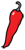The Grand Locus / Life for statistical sciences

the Blog

## Is there a gene for alcoholism? (1)

This is usually the next thing I hear when I say that I am a geneticist. Behind this question and its variants lies a profound and natural interrogration, which could be phrased as "how much of me is the product of my genes?" I made a habit of not answering that question but instead, highlight its inaneness by lecturing people about genetics. So, for once, and exclusively on my blog, here is the tl;dr answer: no, there is not. Now comes the lecture about genetics.

I will start with mental retardation — unrelated with my opinion of those claims, really — and more precisely with the fragile X syndrome. James Watson, the co-discoverer of the structure of DNA and the pioneer of the Human Genome Project declared:

I think it was the first triumph of the Human Genome Project. With fragile X we've got just one protein missing, so it's a simple problem. So, you know, if I were going to work on something with the thought that I were going to solve it, oh boy, I'd work on fragile X.

In other words, there seems to be a gene for mental retardation. The incidence...

## The fallacy of (in)dependenceIn the post Why p-values are crap I argued that independence is a key assumption of statistical testing and that it almost never holds in practical cases, explaining how p-values can be insanely low even in the absence of effect. However, I did not explain how to test independence. As a matter of fact I did not even define independence because the concept is much more complex than it seems.

Apart from the singular case of Bayes theorem, which I referred to in my previous post, the many conflicts of probability theory have been settled by axiomatization. Instead of saying what probabilities are, the current definition says what properties they have. Likewise, independence is defined axiomatically by saying that events $A$ and $B$ are independent if $P(A \cap B) = P(A)P(B)$, or in English, if the probability of observing both is the product of their individual probabilities. Not very intuitive, but if we recall that $P(A|B) = P(A \cap B)/P(B)$, we see that an alternative formulation of the independence of $A$ and $B$ is $P(A | B) = P(A)$. In other words, if $A$ and $B$ are independent, observing...